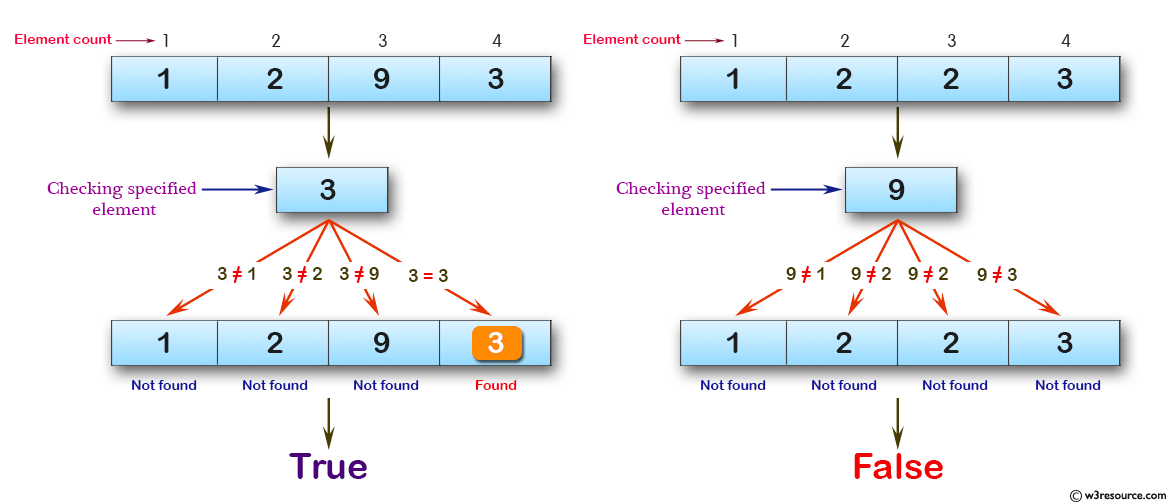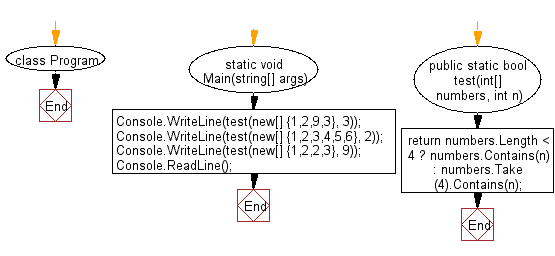﻿ C# - First 4 elements must be equal in an array of integers# C# Sharp Basic Algorithm Exercises: Check whether one of the first 4 elements in an array of integers is equal to a given element

## C# Sharp Basic Algorithm: Exercise-33 with Solution

Write a C# Sharp program to check whether one of the first 4 elements in an array of integers is equal to a given element.

Pictorial Presentation:Sample Solution:-

C# Sharp Code:

``````using System;
using System.Linq;
namespace exercises
{
class Program
{
static void Main(string[] args)
{
Console.WriteLine(test(new[] {1,2,9,3}, 3));
Console.WriteLine(test(new[] {1,2,3,4,5,6}, 2));
Console.WriteLine(test(new[] {1,2,2,3}, 9));
}
public static bool test(int[] numbers, int n)
{
return numbers.Length < 4 ? numbers.Contains(n) : numbers.Take(4).Contains(n);
}
}
}
```
```

Sample Output:

```True
True
False```

Flowchart:C# Sharp Code Editor:

Improve this sample solution and post your code through Disqus

What is the difficulty level of this exercise?

Test your Programming skills with w3resource's quiz.

﻿